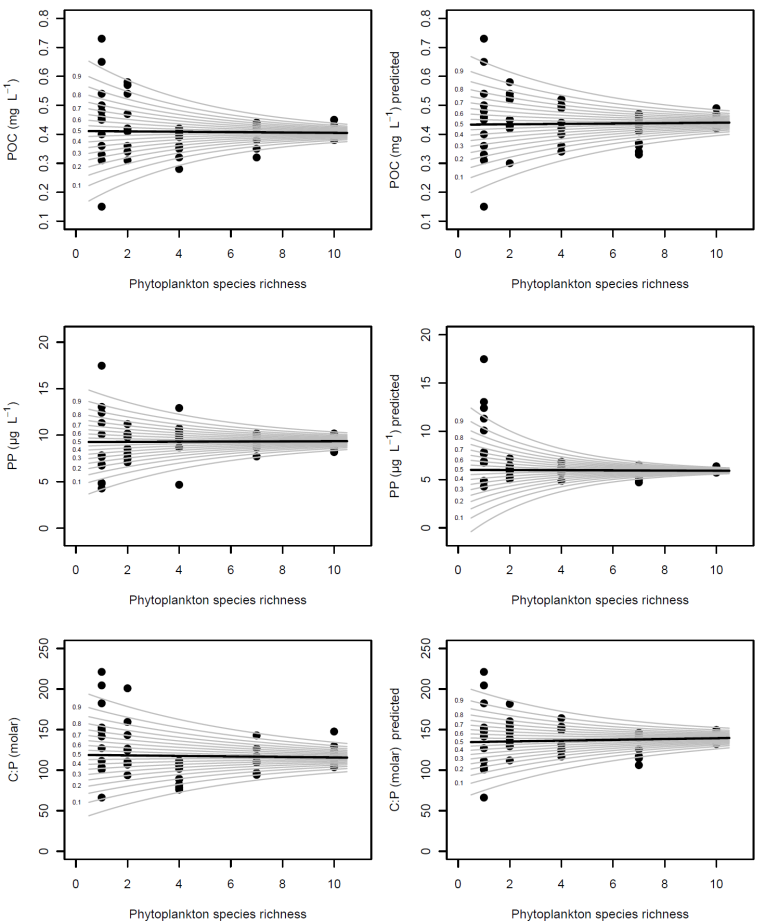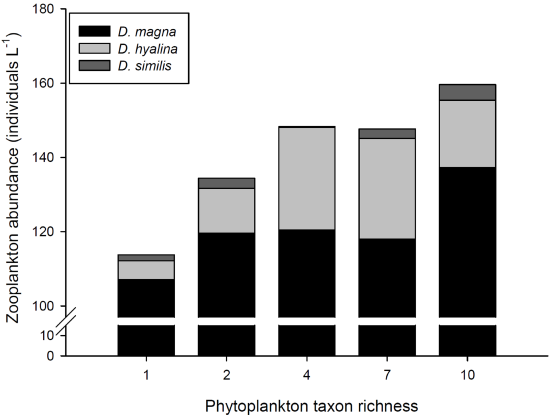Ecological Archives E093-252-A1

Maren Striebel, Gabriel Singer, Herwig Stibor, Tom Andersen. 2012. “Trophic overyielding”: Phytoplankton diversity promotes zooplankton productivity. Ecology 93:2719–2727. http://dx.doi.org/10.1890/12-0003.1

Appendix A. A table summarizing all experimental treatments and main characteristics of the algae used as food for our experiment; a table showing carbon biomass, phosphorus content, and stoichiometry of the phytoplankton communities; a figure showing phytoplankton richness related to POC, POC predicted, PP, PP predicted, C:P, and C:P predicted; and a figure showing average zooplankton community composition at the outcome of the experiment as stacked bar plots for each phytoplankton diversity treatment.

Appendix A. A table summarizing all experimental treatments and main characteristics of the algae used as food for our experiment, a table showing carbon biomass, phosphorous content, and stoichiometry of the phytoplankton communities, a figure showing phytoplankton richness related to POC, POC predicted, PP, PP predicted, C:P, and C:P predicted, and a figure showing average zooplankton community composition at the outcome of the experiment as stacked bar plots for each phytoplankton diversity treatment.

Table A1. Summary of all experimental treatments and main characteristics of the algae used as food for this experiment.

 Species Strain nr Volume μm³ Class Taxon richness 1 Taxon richness 2 Taxon richness 3 Taxon richness 7 Taxon richness 8 1 2 3 4 5 6 7 8 9 10 51 52 11 12 13 14 15 16 17 18 19 20 21 22 23 24 25 26 27 28 29 30 31 32 33 34 35 36 37 38 39 40 41 42 43 44 45 46 47 48 49 50 Chlamydomonas reinhardii SAG 11-31 207.6 Chlorophyta x x x x x x x x x x x x x x x x x x Scenedesmus obliquus SAG 276-10 289.8 x x x x x x x x x x x x x x x x x x x Selenastrum capricornutum UTCC 37 597.9 x x x x x x x x x x x x x x x x x x x x x x x x x Carteria sp. SAG 8-4 1836.0 x x x x x x x x x x x x x x x x x x x x x x x Staurastrum tetracerum SAG 7.94 887.0 x x x x x x x x x x x x x x x x x x x x Haematococcus pluvialis SAG 34-1l 1709.0 x x x x x x x x x x x x x x x x x x Microcystis wesenbergii MPI Plön 84.7 Cyanobacteria x x x x x x x x x x x x x x x x x x Chroococcus minutus SAG 41.79 234.9 x x x x x x x x x x x x x x x x Pseudanabaena galeata SAG 13.83 3199.0 x x x x x x x x x x x x x x x x x x x x x x Cryptomonas phaseolus SAG 2013 799.0 Cryptophyceae x x x x x x x x x x x x x x x x x x x x x x Fragilaria crotonensis SAG 28.96 2022.0 Bacillariophyta x x x x x x x x x x x x x x x x x x x x Nitzschia palea SAG 1052-3a 1095.0 x x x x x x x x x x x x x x x x x x x x x

Table A2. Carbon biomass, phosphorous content and stoichiometry of the phytoplankton communities.

 Equation P n 95% CI POC (mg L-1) 0.41 + 0.0006 SR 0.8 52 13.98–23.40% POC (mg L-1) predicted 0.43 + 0.0007 SR 0.8 52 10.73–21.07% PP (μg L-1) 9.26 + 0.009 SR 0.9 52 11.94–21.10% PP (μg L-1) predicted 6.008 + 0.009 SR 0.8 52 21.98–30.24% C:P (molar) 118.84 + 0.3 SR 0.8 52 8.43–18.46% C:P (molar) predicted 134.34 + 0.5 SR 0.5 52 9.61–20.93%

Quantity (carbon biomass), phosphorous content and stoichiometry of the artificial phytoplankton communities (all data mean values based on three determinations during the experiment, see methods) used as experimental food treatments are not related to their experimentally manipulated species richness. Given are fitted linear models allowing variable variances (GAMLSS models) with phytoplankton species richness (SR) as independent variable for POC (Fig. A1a), POC predicted (Fig. A1b), PP (Fig. A1c), PP predicted (Fig. A1d), C:P (Fig. A1e), and C:P predicted (Fig. A1f). The column headed by "P" reports probabilities for the trend in the mean across the SR gradient; relationships were never significant. The last column refers to the 95% confidence interval for the relative decrease in variance per phytoplankton species added (i.e. the log-linear slope of the GAMLSS model for the variance); variance decreased significantly with SR in all models.Fig. A1. Phytoplankton richness related to POC (A), POC predicted (B), PP (C), PP predicted (D). C:P (E), and C:P predicted (F).

All data shown are mean values based on three determinations during the experiment and were fitted with GAMLSS models where the heavy black line represents the conditional expectation and gray lines represent percentiles of the conditional distribution. See Table A2 for parameter estimates of the fitted models.Fig. A2. Average zooplankton community composition at the outcome of the experiment as stacked bar plots for each phytoplankton diversity treatment.

Higher abundances of D. magna might be due to higher body size and thus higher numbers of juvenile offspring.